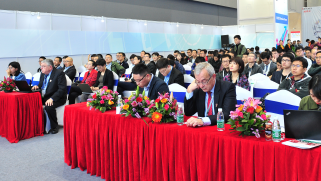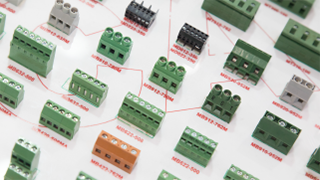# 电缆的种类

电缆产品的种类有成千上万，应用在各行各业中。

1、裸线类

2、电磁线（漆包线）

3、电力电缆

4、电气装备用电线电缆（电气装备用绝缘电线，公路车辆用电线，电机绕组线，航空电线，矿用电缆，船用电缆，石油及地质用电缆，电梯电缆，控制信号电缆，等等等，这类电缆种类繁多）

5、通信电线电缆与光缆

1． 铜材的成本

2． PVC（外边料皮）的成本

3． 人工的成本

1.772 / 2=0.886那面积就=3.14*0.886*0.886 =2.46这个值指的就是平方毫米。之所以BV2.5平方电

2.189*今日铜价(73)=159.8元

159.8元就是铜的原材料成本

PVC外面材料的成本

8 10 15  10 14 18

1。导体用量：（Kg/Km）=d2 × 0.7854 × G × N × K1 × K2 × C

d=铜线径 G=铜比重 N=条数 K1=铜线绞入率 K2=芯线绞

2。绝缘用量：（Kg/Km）=（D2 - d2）× 0.7854 × G × C × K2

D=绝缘外径 d=导体外径 G=绝缘比重 K2=芯线绞入率 C=绝缘芯线根数

3。外被用量：（Kg/Km）= ( D12 - D2 ) × 0.7854 × G

D1=完成外径 D=上过程外径 G=绝缘比重

4。包带用量：（Kg/Km）= D2 × 0.7854 × t × G × Z

D=上过程外径 t=包带厚度 G=包带比重 Z=重叠率(1/4Lap = 1.25)。

5。缠绕用量：（Kg/Km）= d2 × 0.7854 × G × N × Z

d=铜线径 N=条数 G=比重 Z=绞入率

6。编织用量：（Kg/Km）= d2 × 0.7854 × T × N × G / cosθ

θ = atan( 2 × 3.1416 × ( D + d × 2 )) × 目数 / 25.4 / T

d=编织铜线径 T=锭数 N=每锭条数 G=铜比重

PVC-1.45；LDPE-0.92；HDPE-0.96；PEF（发泡）-0.65；FRPE-1.7；Teflon（FEP）2.2；Nylon-0.97；PP-0.97；PU-1.21

### 主办单位官方微信